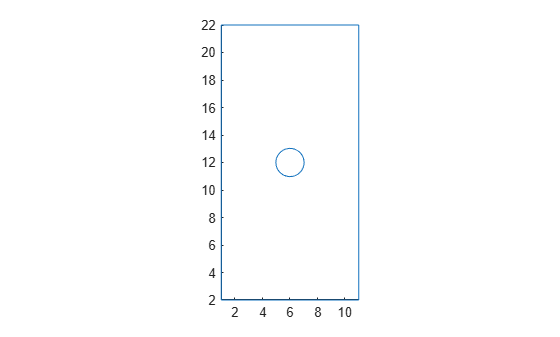translate

Translate geometry

Description

example

translate(g,s) translates the geometry g by the distance s.

h = translate(g,s) returns a handle h to the resulting geometry object g. If the original geometry is a DiscreteGeometry object, then the function modifies the original geometry and returns the handle h to the modified DiscreteGeometry geometry object. If the original geometry is an AnalyticGeometry object, then h is a handle to a new DiscreteGeometry geometry object. In this case, the original geometry remains unchanged.

Examples

collapse all

Translate a geometry by different distances along the x- and y-axes.

Create a model.

model = createpde;

Import and plot a geometry.

g = importGeometry(model,'PlateHolePlanar.stl');
pdegplot(g)Mesh the geometry and plot the mesh.

generateMesh(model);

figure
pdemesh(model)Translate the 2-D geometry by 1 along the x-axis and by 2 along the y-axis. Plot the result.

translate(g,[1 2]);
pdegplot(g)Plot the geometry and mesh. The translate function modifies a geometry, but it does not modify a mesh.

figure
pdegplot(g)
hold on
pdemesh(model)After modifying the geometry, always regenerate the mesh.

generateMesh(model);

figure
pdegplot(g)
hold on
pdemesh(model)Input Arguments

collapse all

Geometry, specified as a DiscreteGeometry or AnalyticGeometry object.

Translation distance, specified as a vector of two or three real numbers. The distance is a vector of two elements for a 2-D geometry or three elements for a 3-D geometry. The elements specify the distance along the x-, y-, and, for a 3-D geometry, z-axes.

Output Arguments

collapse all

Resulting geometry, returned as a handle. If the original geometry g is a DiscreteGeometry object, then h is a handle to the modified DiscreteGeometry geometry object g. If g is an AnalyticGeometry object, then h is a handle to a new DiscreteGeometry geometry object. In this case, the original geometry g remains unchanged.

Tips

• translate modifies a geometry, but it does not modify a mesh. After modifying a geometry, regenerate the mesh to ensure a proper mesh association with the new geometry.

• If g is an AnalyticGeometry object, and you want to replace it with the resulting discrete geometry, assign the output to the original geometry, for example, g = translate(g,[1 2]).